UIHistories Project: A History of the University of Illinois by Kalev Leetaru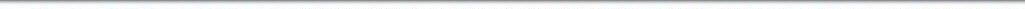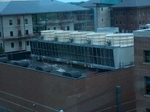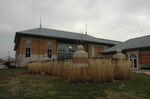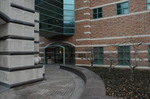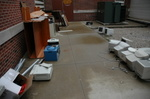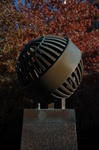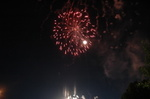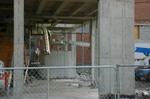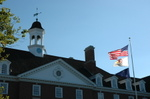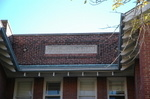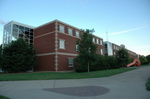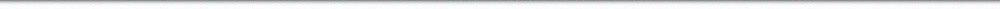N A V I G A T I O N D I G I T A L L I B R A R Y

## Repository: UIHistories Project: Board of Trustees Minutes - 1896 [PAGE 207]

Caption: Board of Trustees Minutes - 1896
This is a reduced-resolution page image for fast online browsing.

< Previous Page [Displaying Page 207 of 371] Next Page >
[VIEW ALL PAGE THUMBNAILS]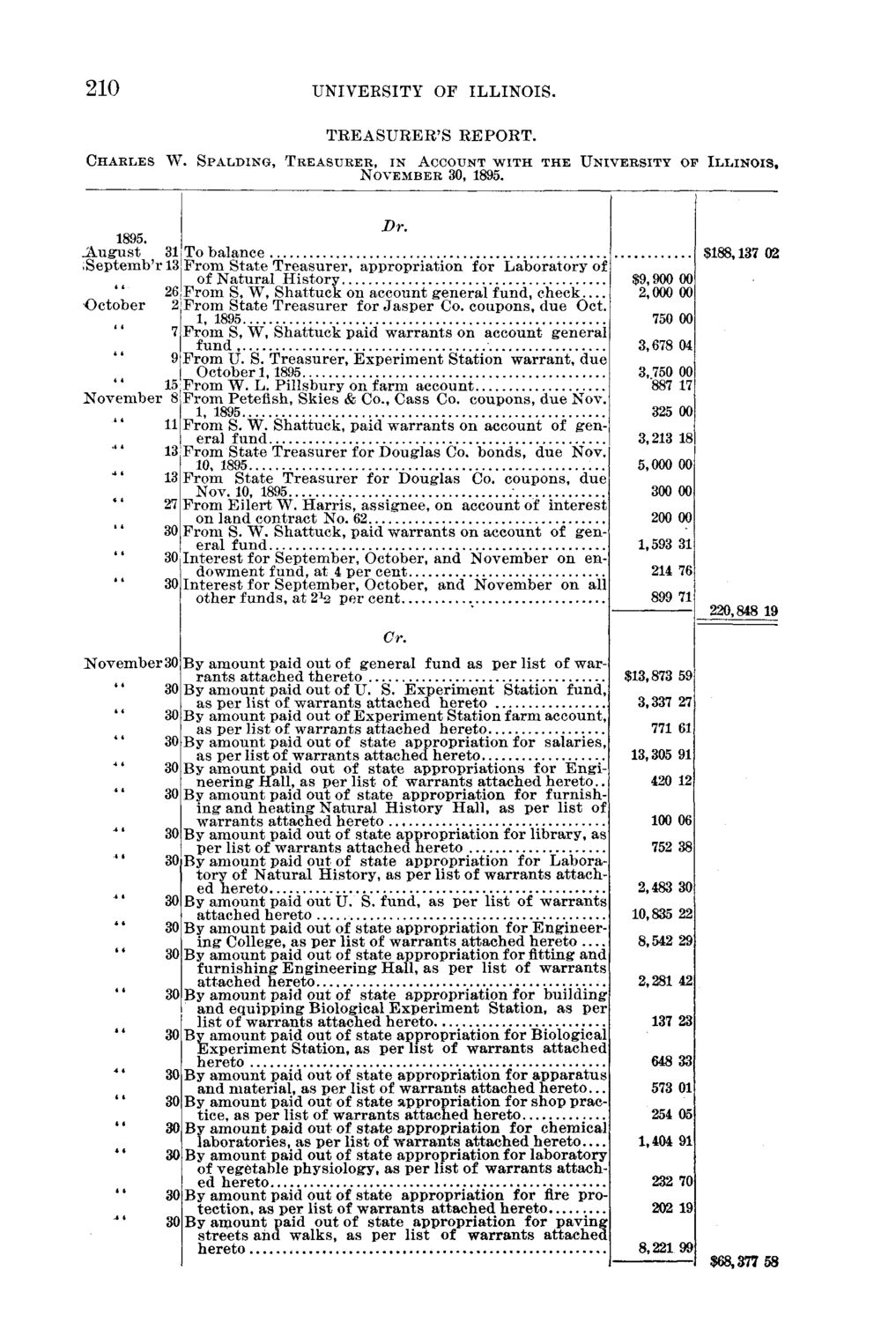### EXTRACTED TEXT FROM PAGE:

210

C H A R L E S W.

UNIVERSITY OF ILLINOIS. TREASURER'S REPORT.

S P A L D I N G , T R E A S U R E R , I N ACCOUNT W I T H T H E U N I V E R S I T Y O F I L L I N O I S , N O V E M B E R 30, 1895.

Dr. 1895. .August 31 To balance .. ;Septemb'r 131 F r o m State Treasurer, appropriation for Laboratory of of N a t u r a l History 26 F r o m S, W, Shattuck on account general fund, check October 2| F r o m State T r e a s u r e r for J a s p e r Co. coupons, due Oct. 1, 1895 7 F r o m S, W, S h a t t u c k paid w a r r a n t s on account general fund 9| F r o m U. S. Treasurer, E x p e r i m e n t Station warrant, d u e October 1,1895 15 F r o m W. L. Pillsbury on farm account N o v e m b e r 8| F r o m Peteflsh, Skies & Co., Cass Co. coupons, due Nov. 1, 1895. 11 F r o m S. W. Shattuck, paid w a r r a n t s on account of general fund 13 F r o m State T r e a s u r e r for Douglas Co. bonds, due Nov. 10, 1895 13 F r o m State T r e a s u r e r for Douglas Co. coupons, d u e Nov. 10, 1895 ; 27 F r o m Eilert W. H a r r i s , assignee, on account of interest| on land contract No. 62 30 F r o m S. W. Shattuck, paid w a r r a n t s on account of general fund 301 I n t e r e s t for September, October, and November on endowment fund, at 4 per cent 30 I n t e r e s t for September, October, and November on all other funds, at 2 ^ p e r cent Cr.

\$188,137 02 \$9,900 00 2,000 001 750 OOJ

3,678 04 3,750 00 "887 17 325 00 3,213 181 5,000 00 300 00 200 001 1,593 31 214 761 899 71

220,848 19

N o v e m b e r 30 By amount paid out of general fund as p e r list of war\$13,873 59 r a n t s attached thereto 30 By amount paid out of U. S. E x p e r i m e n t Station fund,| 3,337 27] as per list of w a r r a n t s attached hereto I 30 By amount paid out of E x p e r i m e n t Station farm account, 771 61 as p e r list of w a r r a n t s attached hereto j 30 By amount paid out of state appropriation for salaries,} 13,305 91; as per list of w a r r a n t s attached hereto 30 By amount paid out of state appropriations for Engi- 1 420 12; n e e r i n g Hall, as p e r list of w a r r a n t s attached h e r e t o . . 30 By amount paid out of state appropriation for furnish-l ing and h e a t i n g N a t u r a l H i s t o r y Hall, as p e r list of | 100 06 w a r r a n t s attached hereto 30l By amount paid out of state appropriation for library, as p e r list of w a r r a n t s attached hereto 752 381 30i By amount paid out of state appropriation for Labora-| tory of N a t u r a l History, as p e r list of w a r r a n t s attached hereto 2,483 30 By amount paid out U. S. fund, as p e r list of w a r r a n t s 10,835 22 attached hereto By amount paid out of state appropriation for Engineer8,542 29 ing College, as p e r list of w a r r a n t s attached hereto — By amount paid out of state appropriation for fitting and furnishing E n g i n e e r i n g Hall, as p e r list of w a r r a n t s attached hereto 2,281 42 By amount paid out of state appropriation for building and equipping Biological E x p e r i m e n t Station, as per| list of w a r r a n t s attached hereto 137 23 By amount paid out of state appropriation for Biological E x p e r i m e n t Station, as p e r list of w a r r a n t s attached hereto 648 33) By amount paid out of state appropriation for a p p a r a t u s and material, as p e r list of w a r r a n t s attached h e r e t o . 573 01 By amount paid out of state appropriation for shop prac-| tice, as per list of w a r r a n t s attached hereto 254 051 By a m o u n t paid out of state appropriation for chemical laboratories, as p e r list of w a r r a n t s attached h e r e t o — 1,404 91 By amount paid out of state appropriation for laboratory of vegetable physiology, as p e r list of w a r r a n t s attach ' ed hereto — 232 70| By amount paid out of state appropriation for fire pro202 19 tection, as per list of w a r r a n t s attached hereto By amount paid out of state appropriation for p a v i n g streets and walks, as per list of w a r r a n t s attached| hereto 8,221 99 \$68,377 58